# Portfolio Backtesting

### 2022-04-20

This vignette illustrates the usage of the package portfolioBacktest for automated portfolio backtesting over multiple datasets on a rolling-window basis. It can be used by a researcher/practitioner to backtest a set of different portfolios, as well as a course instructor to assess the students in their portfolio design in a fully automated and convenient manner. The results can be nicely formatted in tables and plots.

# Package Snapshot

Backtesting is a dangerous task fraught with many potential pitfalls (Luo et al. 2014). By performing a large number of randomized backtests, instead of visually inspecting a single backtest, one can obtain more realistic results.

This package backtests a list of portfolios over multiple datasets on a rolling-window basis (aka walk forward), producing final results as in the following.

• Performance table:

• Barplot: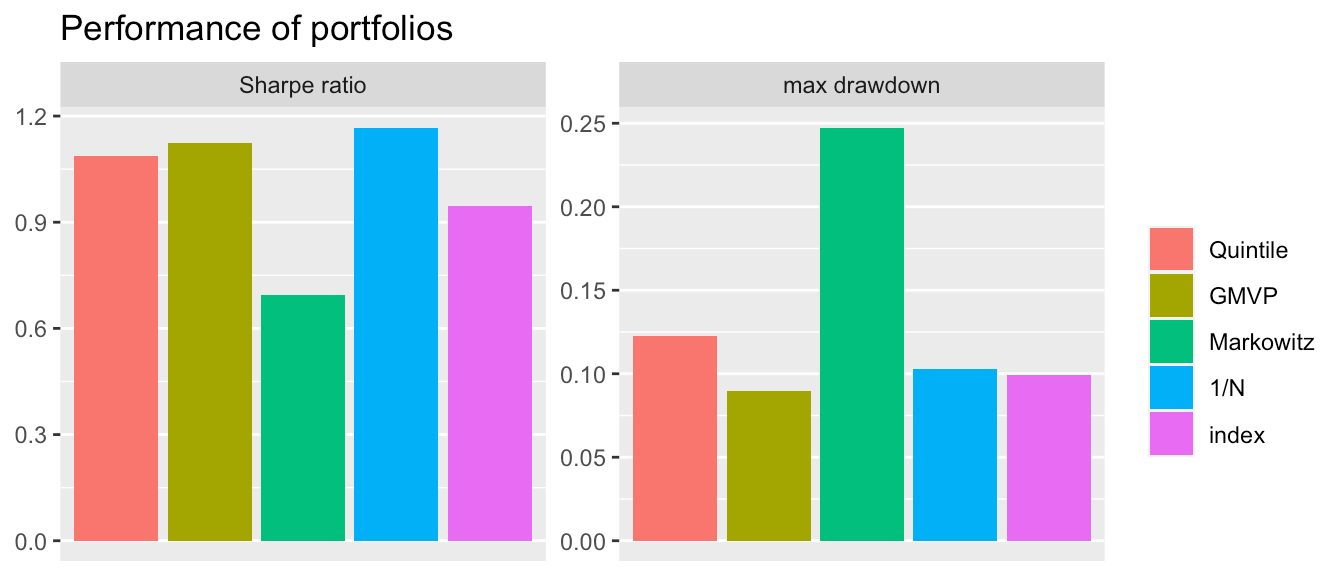• Boxplot: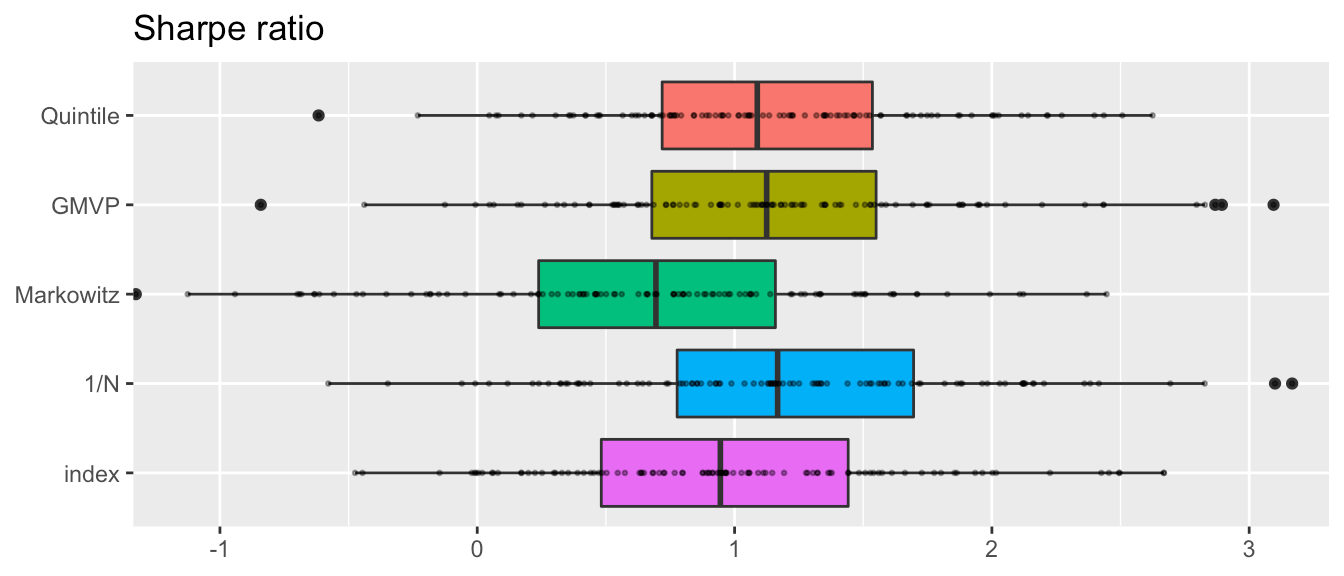# Quick Start

Do the backtest on your own portfolio following few steps:

• Step 1 - load package & 10 datasets
library(portfolioBacktest)
data("dataset10")
• Step 2 - define your own portfolio
my_portfolio <- function(dataset, ...) {
prices <- dataset$adjusted N <- ncol(prices) return(rep(1/N, N)) } • Step 3 - do backtest bt <- portfolioBacktest(my_portfolio, dataset10) #> Backtesting 1 portfolios over 10 datasets (periodicity = daily data)... • Step 4 - check your portfolio performance (e.g., median of the 10 individual backtests) backtestSummary(bt)$performance
#>                            fun1
#> Sharpe ratio       1.476203e+00
#> max drawdown       8.937890e-02
#> annual return      1.594528e-01
#> annual volatility  1.218623e-01
#> Sortino ratio      2.057677e+00
#> downside deviation 8.351402e-02
#> Sterling ratio     2.122653e+00
#> Omega ratio        1.295090e+00
#> VaR (0.95)         1.101934e-02
#> CVaR (0.95)        1.789425e-02
#> rebalancing period 1.000000e+00
#> turnover           8.641594e-03
#> ROT (bps)          7.334458e+02
#> cpu time           1.615385e-03
#> failure rate       0.000000e+00

# Installation

The package can be installed from CRAN or GitHub:

# install stable version from CRAN
install.packages("portfolioBacktest")

# install development version from GitHub
devtools::install_github("dppalomar/portfolioBacktest")

# Getting help
library(portfolioBacktest)
help(package = "portfolioBacktest")
?portfolioBacktest

## Basic structure of datasets

The main function portfolioBacktest() requires the argument dataset_list to follow a certain format: it should be a list of several individual datasets, each of them being a list of several xts objects following exactly the same date index. One of those xts objects must contain the historical prices of the stocks, but we can have additional xts objects containing other information such as volume of the stocks or index prices. The package contains a small dataset sample for illustration purposes:

data("dataset10")  # load the embedded dataset
class(dataset10)  # show dataset class
#>  "list"
names(dataset10[1:3])  # show names of a few datasets
#>  "dataset 1" "dataset 2" "dataset 3"
names(dataset10$dataset 1) # structure of one dataset #>  "adjusted" "index" head(dataset10$dataset 1$adjusted[, 1:3]) #> MAS.Adjusted MGM.Adjusted CMI.Adjusted #> 2015-04-24 22.05079 21.34297 121.8492 #> 2015-04-27 22.13499 21.26537 124.3041 #> 2015-04-28 22.68226 21.69223 122.5187 #> 2015-04-29 22.54755 20.47956 123.2150 #> 2015-04-30 22.30337 20.51836 123.4203 #> 2015-05-01 22.85065 20.76090 125.9823 Note that each dataset contains an xts object called "adjusted" (adjusted prices). By default, portfolioBacktest() will use such adjusted prices to calculate the portfolio return. But one can change this setting with the argument price_name in function portfolioBacktest(). ## Obtaining more data We emphasize that 10 datasets are not enough for properly backtesting portfolios. In this package, we provide the function stockDataDownload() to download online data resources in the required data format. Then, the function financialDataResample() can help resample the downloaded data into multiple datasets (each resample is obtained by randomly choosing a subset of the stock names and randomly choosing a time period over the available long period), which can be directly passed to portfolioBacktest(). We recommend using these two functions to generate multiple datasets for serious backtesting: data(SP500_symbols) # load the SP500 symbols # download data from internet SP500 <- stockDataDownload(stock_symbols = SP500_symbols, from = "2008-12-01", to = "2018-12-01") # resample 10 times from SP500, each with 50 stocks and 2-year consecutive data my_dataset_list <- financialDataResample(SP500, N_sample = 50, T_sample = 252*2, num_datasets = 10) Each individual dataset will contain 7 xts objects with names: open, high, low, close, volume, adjusted, index. Since the function stockDataDownload() may take a long time to download the data from the Internet, it will automatically save the data into a local file for subsequent fast retrieval (whenever the function is called with the same arguments). It is the responsibility of the user to download a proper universe of stocks to avoid survivorship bias. ## Expanding the datasets Additional data can be helpful in designing portfolios. One can add as many other xts objects in each dataset as desired. For example, if the Moving Average Convergence Divergence (MACD) information is needed by the portfolio functions, one can manually add it to the dataset as follows: for (i in 1:length(dataset10)) dataset10[[i]]$MACD <- apply(dataset10[[i]]$adjusted, 2, function(x) { TTR::MACD(x)[ , "macd"] }) # Defining Portfolios A portfolio has to be defined in the form of function that takes as input: 1. a dataset (which will be automatically windowed during the backtesting following a rolling-window basis) containing a list of xts objects (following the format of the elements of the argument dataset_list) and 2. the current portfolio w_current (if this argument is not used, then alternatively one can use the ellipsis ... in the function definition). The portfolio function has to return the portfolio as a numerical vector of normalized weights of the same length as the number of stocks. Below we give the examples for the quintile portfolio, the global minimum variance portfolio (GMVP), and the Markowitz mean-variance portfolio (under practical constraints $$\mathbf{w} \ge \mathbf{0}$$ and $$\mathbf{1}^{T} \mathbf{w} =1$$): # define quintile portfolio quintile_portfolio_fun <- function(dataset, w_current) { X <- diff(log(dataset$adjusted))[-1]  # compute log returns
N <- ncol(X)
# design quintile portfolio
ranking <- sort(colMeans(X), decreasing = TRUE, index.return = TRUE)$ix w <- rep(0, N) w[ranking[1:round(N/5)]] <- 1/round(N/5) return(w) } # define GMVP (with heuristic not to allow shorting) GMVP_portfolio_fun <- function(dataset, ...) { X <- diff(log(dataset$adjusted))[-1]  # compute log returns
Sigma <- cov(X)  # compute SCM
# design GMVP
w <- solve(Sigma, rep(1, nrow(Sigma)))
w <- abs(w)/sum(abs(w))
return(w)
}

# define Markowitz mean-variance portfolio
library(CVXR)
Markowitz_portfolio_fun <- function(dataset, ...) {
X <- diff(log(dataset$adjusted))[-1] # compute log returns mu <- colMeans(X) # compute mean vector Sigma <- cov(X) # compute the SCM # design mean-variance portfolio w <- Variable(nrow(Sigma)) prob <- Problem(Maximize(t(mu) %*% w - 0.5*quad_form(w, Sigma)), constraints = list(w >= 0, sum(w) == 1)) result <- solve(prob) return(as.vector(result$getValue(w)))
}

The argument w_current can be used to control the transaction cost:

Markowitz_portfolio_tc_fun <- function(dataset, w_current) {
tau <- 0.01
X <- diff(log(dataset$adjusted))[-1] # compute log returns mu <- colMeans(X) # compute mean vector Sigma <- cov(X) # compute the SCM # design mean-variance portfolio w <- Variable(nrow(Sigma)) prob <- Problem(Maximize(t(mu) %*% w - 0.5*quad_form(w, Sigma) - tau*sum(abs(w - w_current))), constraints = list(w >= 0, sum(w) == 1)) result <- solve(prob) return(as.vector(result$getValue(w)))
}

# Backtesting and Plotting

## Backtesting your portfolios

With the datasets and portfolios ready, we can now do the backtest easily. For example, to obtain the three portfolios’ performance over the datasets, we just need combine them in a list and run the backtest in one line:

portfolios <- list("Quintile"  = quintile_portfolio_fun,
"GMVP"      = GMVP_portfolio_fun,
"Markowitz" = Markowitz_portfolio_fun)
bt <- portfolioBacktest(portfolios, dataset10, benchmark = c("1/N", "index"))
#> Backtesting 3 portfolios over 10 datasets (periodicity = daily data)...
#> Backtesting benchmarks...

## Result format

Here bt is a list storing all the backtest results according to the passed functions list (plus the two benchmarks):

names(bt)
#>  "Quintile"  "GMVP"      "Markowitz" "1/N"       "index"

Each element of bt is also a list storing more information for each of the datasets:

#>                           levelName
#> 1  bt
#> 2   ¦--Quintile
#> 3   ¦   ¦--dataset 1
#> 4   ¦   ¦   ¦--performance
#> 5   ¦   ¦   ¦--cpu_time
#> 6   ¦   ¦   ¦--error
#> 7   ¦   ¦   ¦--error_message
#> 8   ¦   ¦   ¦--w_optimized
#> 9   ¦   ¦   ¦--w_rebalanced
#> 10  ¦   ¦   ¦--w_bop
#> 11  ¦   ¦   ¦--return
#> 12  ¦   ¦   ¦--wealth
#> 13  ¦   ¦   °--X_lin
#> 14  ¦   ¦--dataset 2
#> 15  ¦   ¦   ¦--performance
#> 16  ¦   ¦   ¦--cpu_time
#> 17  ¦   ¦   ¦--error
#> 18  ¦   ¦   ¦--error_message
#> 19  ¦   ¦   ¦--w_optimized
#> 20  ¦   ¦   °--... 5 nodes w/ 0 sub
#> 21  ¦   °--... 8 nodes w/ 85 sub
#> 22  °--... 4 nodes w/ 533 sub

One can extract any desired backtest information directly from the returned variable bt.

## Shaping your results

The package also contains several convenient functions to extract information from the backtest results.

• Select several performance measures of one specific portfolio:
# select sharpe ratio and max drawdown performance of Quintile portfolio
backtestSelector(bt, portfolio_name = "Quintile",
measures = c("Sharpe ratio", "max drawdown"))
#> $performance #> Sharpe ratio max drawdown #> dataset 1 1.06419447 0.09384104 #> dataset 2 1.22091716 0.10406013 #> dataset 3 2.24635921 0.06952085 #> dataset 4 1.44699083 0.09921398 #> dataset 5 0.08849001 0.17328255 #> dataset 6 0.99108926 0.10320105 #> dataset 7 1.64175055 0.08836202 #> dataset 8 -0.10916655 0.27460141 #> dataset 9 1.62468886 0.11288730 #> dataset 10 1.37221717 0.09834212 • Tables of several performance measures of the portfolios (classified by performance criteria): # show the portfolios performance in tables backtestTable(bt, measures = c("Sharpe ratio", "max drawdown")) #>$Sharpe ratio
#>               Quintile       GMVP    Markowitz       1/N      index
#> dataset 1   1.06419447 1.32027278  0.001373386 1.4089909 1.33623612
#> dataset 2   1.22091716 0.16541826  1.044875366 0.4355269 0.22256998
#> dataset 3   2.24635921 1.87705877  1.149650946 2.2566129 1.79107233
#> dataset 4   1.44699083 1.12233673  0.331281647 1.2145246 0.95372278
#> dataset 5   0.08849001 0.05190026  0.061763031 0.3137457 0.20553014
#> dataset 6   0.99108926 2.08192072  0.767195436 1.7823589 2.49533696
#> dataset 7   1.64175055 2.69917968 -0.251919230 2.3238009 1.58760559
#> dataset 8  -0.10916655 0.16661653  1.075091650 0.1975010 0.03506698
#> dataset 9   1.62468886 1.27456766  0.779205761 1.5434145 1.37981616
#> dataset 10  1.37221717 1.96674860  0.483356334 1.8760481 1.72522587
#>
#> $max drawdown #> Quintile GMVP Markowitz 1/N index #> dataset 1 0.09384104 0.05733409 0.20824654 0.06678369 0.05595722 #> dataset 2 0.10406013 0.13027178 0.23314406 0.13218930 0.12352525 #> dataset 3 0.06952085 0.04947330 0.16813539 0.04800769 0.05761261 #> dataset 4 0.09921398 0.10466982 0.15162953 0.10861575 0.10159531 #> dataset 5 0.17328255 0.08719220 0.64819903 0.11546985 0.10159531 #> dataset 6 0.10320105 0.02596655 0.33947368 0.03869864 0.02796792 #> dataset 7 0.08836202 0.05441919 0.27695995 0.05440115 0.07671058 #> dataset 8 0.27460141 0.16788147 0.28835512 0.19664607 0.17904681 #> dataset 9 0.11288730 0.10417538 0.24967353 0.10097014 0.10159531 #> dataset 10 0.09834212 0.05701569 0.09785333 0.07778765 0.05595722 • Summary of performance measures: res_sum <- backtestSummary(bt) names(res_sum) #>  "performance_summary" "error_message" res_sum$performance_summary
#>                        Quintile         GMVP    Markowitz          1/N        index
#> Sharpe ratio         1.29656717 1.297420e+00   0.62527589 1.476203e+00   1.35802614
#> max drawdown         0.10120752 7.226314e-02   0.24140880 8.937890e-02   0.08915294
#> annual return        0.19595757 1.441586e-01   0.20402853 1.594528e-01   0.14822709
#> annual volatility    0.16224595 1.107614e-01   0.31015587 1.218623e-01   0.12422862
#> Sortino ratio        1.90905709 1.815420e+00   0.85264675 2.057677e+00   1.90434670
#> downside deviation   0.11132931 7.959349e-02   0.21019793 8.351402e-02   0.08843501
#> Sterling ratio       1.92937203 1.954336e+00   1.20389040 2.122653e+00   2.02000933
#> Omega ratio          1.24641783 1.257818e+00   1.12755743 1.295090e+00   1.28610811
#> VaR (0.95)           0.01608051 9.641547e-03   0.02896669 1.101934e-02   0.01228986
#> CVaR (0.95)          0.02387980 1.669202e-02   0.04233197 1.789425e-02   0.01937577
#> rebalancing period   1.00000000 1.000000e+00   1.00000000 1.000000e+00 252.00000000
#> turnover             0.02944171 2.131205e-02   0.03293795 8.641594e-03   0.00000000
#> ROT (bps)          254.70011596 2.304439e+02 195.65068681 7.334458e+02           NA
#> cpu time             0.00200000 2.346154e-03   0.22757692 1.538462e-03   0.00100000
#> failure rate         0.00000000 0.000000e+00   0.00000000 0.000000e+00   0.00000000

For more flexible usage of these functions, one can refer to the help pages of these functions.

## Plotting your results

Besides, the package also provides some functions to show results in tables and figures.

• Performance table:
summaryTable(res_sum, type = "DT", order_col = "Sharpe ratio", order_dir = "desc")

• Barplot (provides information from summaryTable() in a visual way):
summaryBarPlot(res_sum, measures = c("Sharpe ratio", "max drawdown"))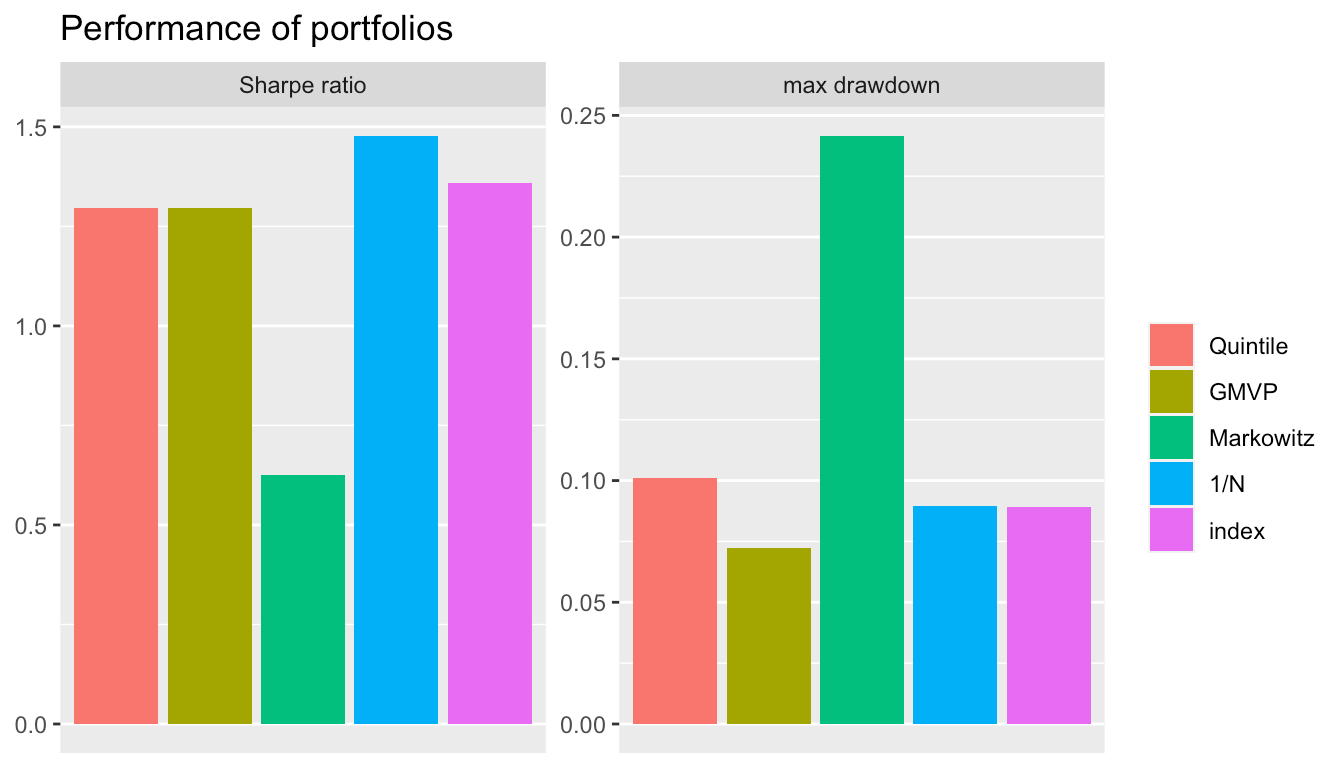• BoxPlot (probably the best way to properly compare the performance of different portfolios with a single performance measure):
backtestBoxPlot(bt, measure = "Sharpe ratio")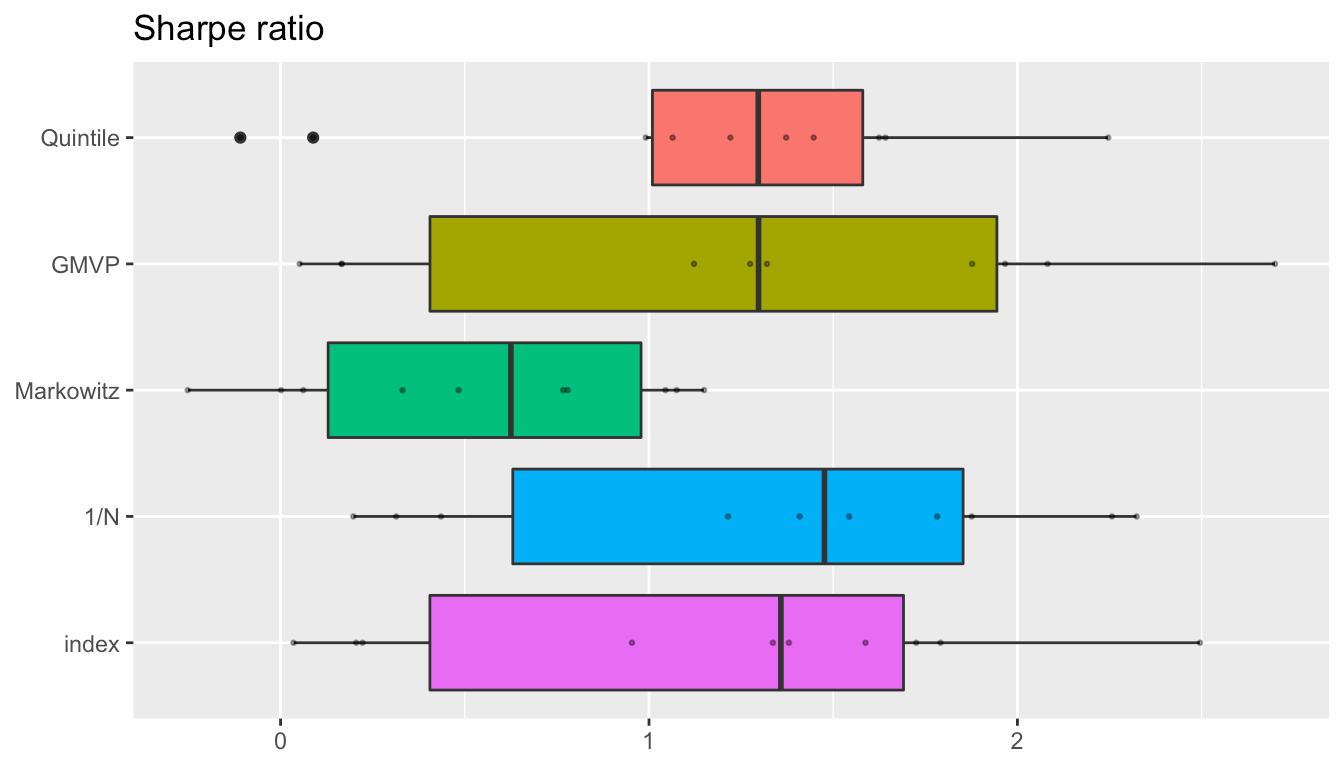• Cumulative return or wealth plot of a single backtest:
backtestChartCumReturn(bt, c("Quintile", "GMVP", "index"))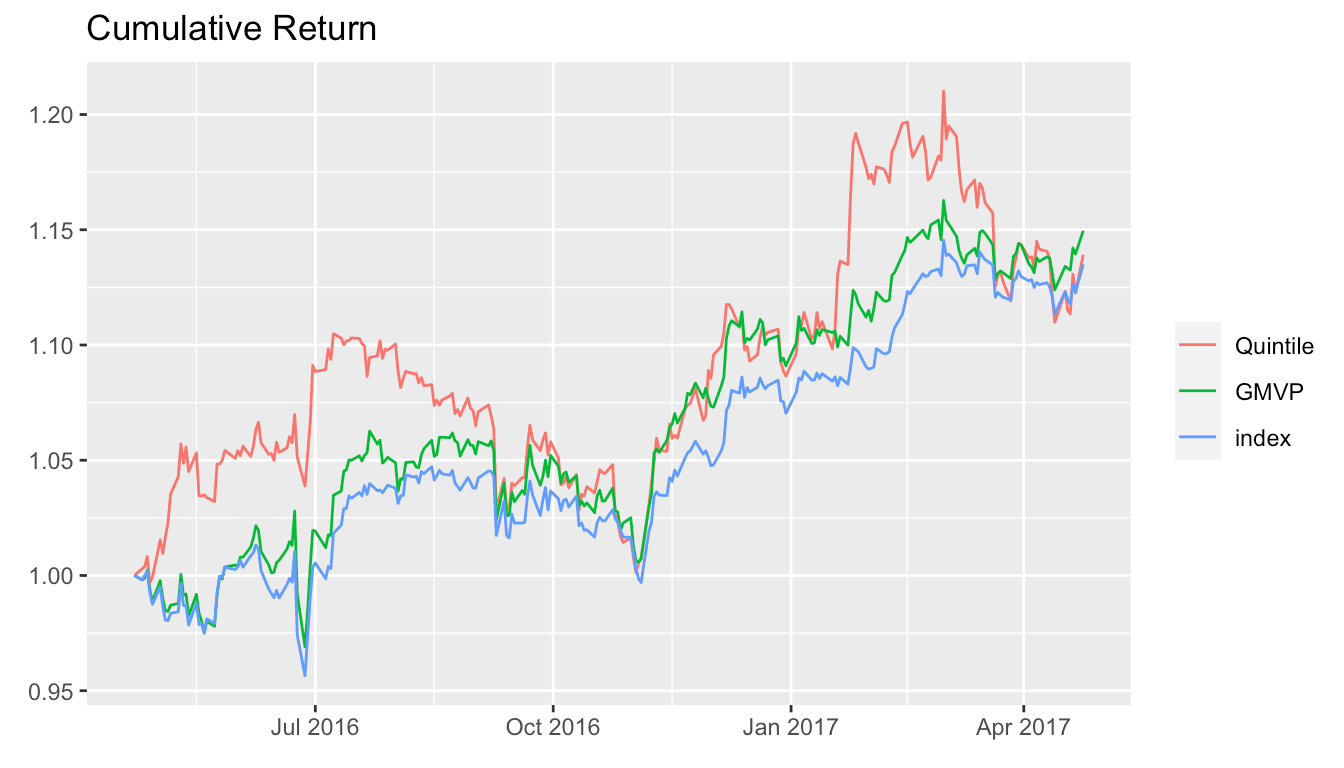backtestChartDrawdown(bt, c("Quintile", "GMVP", "index"))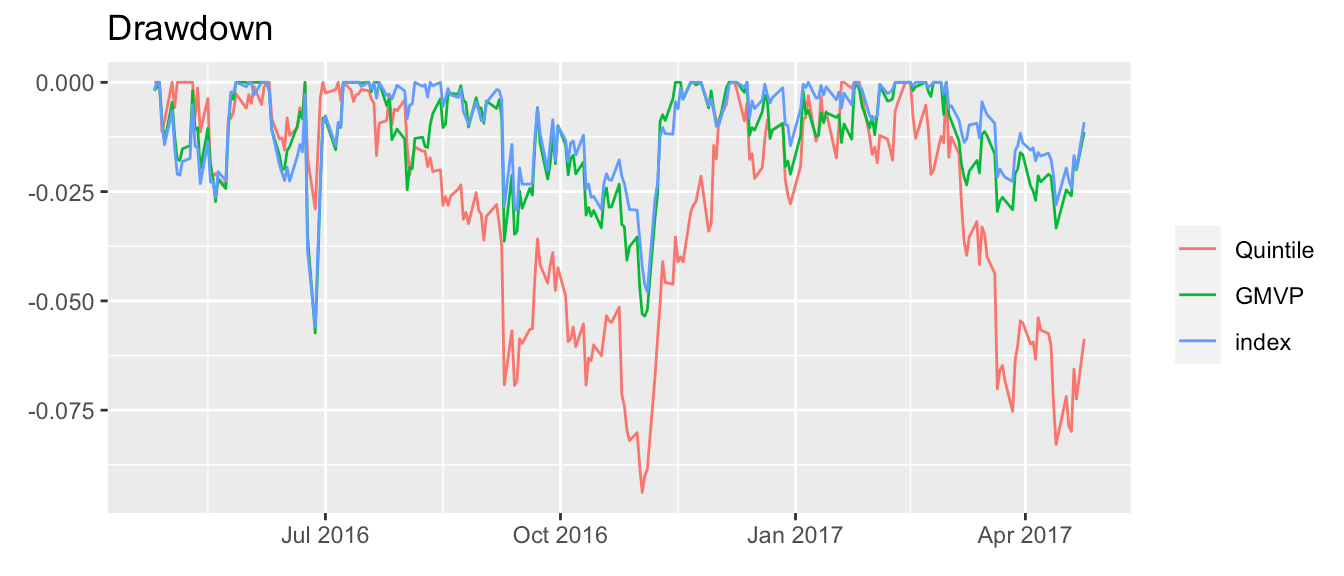• Portfolio allocation evolution of a particular portfolio over a particular backtest:
# for better illustration, let's use only the first 5 stocks
dataset10_5stocks <- lapply(dataset10,
function(x) {x$adjusted <- x$adjusted[, 1:5]; return(x)})
# backtest
bt <- portfolioBacktest(list("GMVP" = GMVP_portfolio_fun), dataset10_5stocks,
rebalance_every = 20)
#> Backtesting 1 portfolios over 10 datasets (periodicity = daily data)...

# chart
backtestChartStackedBar(bt, "GMVP", legend = TRUE)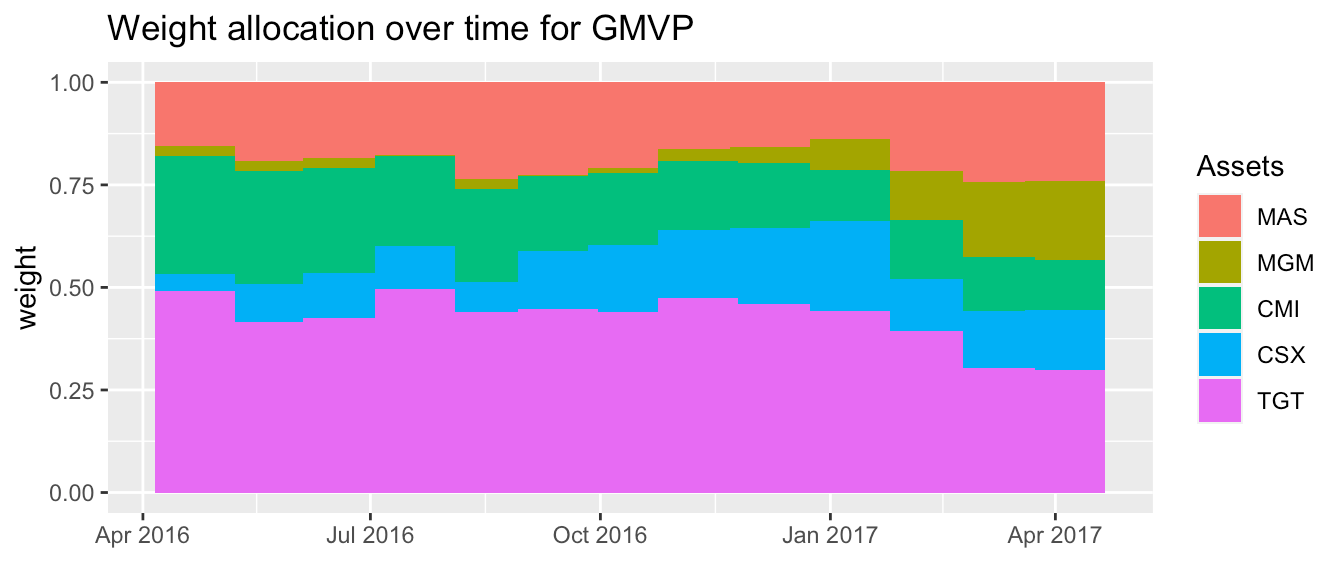## Transaction costs

By default, transaction costs are not included in the backtesting, but the user can easily specify the cost to be used for a more realistic backtesting:

library(ggfortify)

# backtest without transaction costs
bt <- portfolioBacktest(my_portfolio, dataset10)

# backtest with costs of 15 bps
bt_tc <- portfolioBacktest(my_portfolio, dataset10,
cost = list(buy = 15e-4, sell = 15e-4))

# plot wealth time series
wealth <- cbind(bt$fun1$dataset 1$wealth, bt_tc$fun1$dataset 1$wealth)
colnames(wealth) <- c("without transaction costs", "with transaction costs")

autoplot(wealth, facets = FALSE, main = "Wealth") +
theme(legend.title = element_blank()) +
theme(legend.position = c(0.8, 0.2)) +
scale_color_manual(values = c("red", "black"))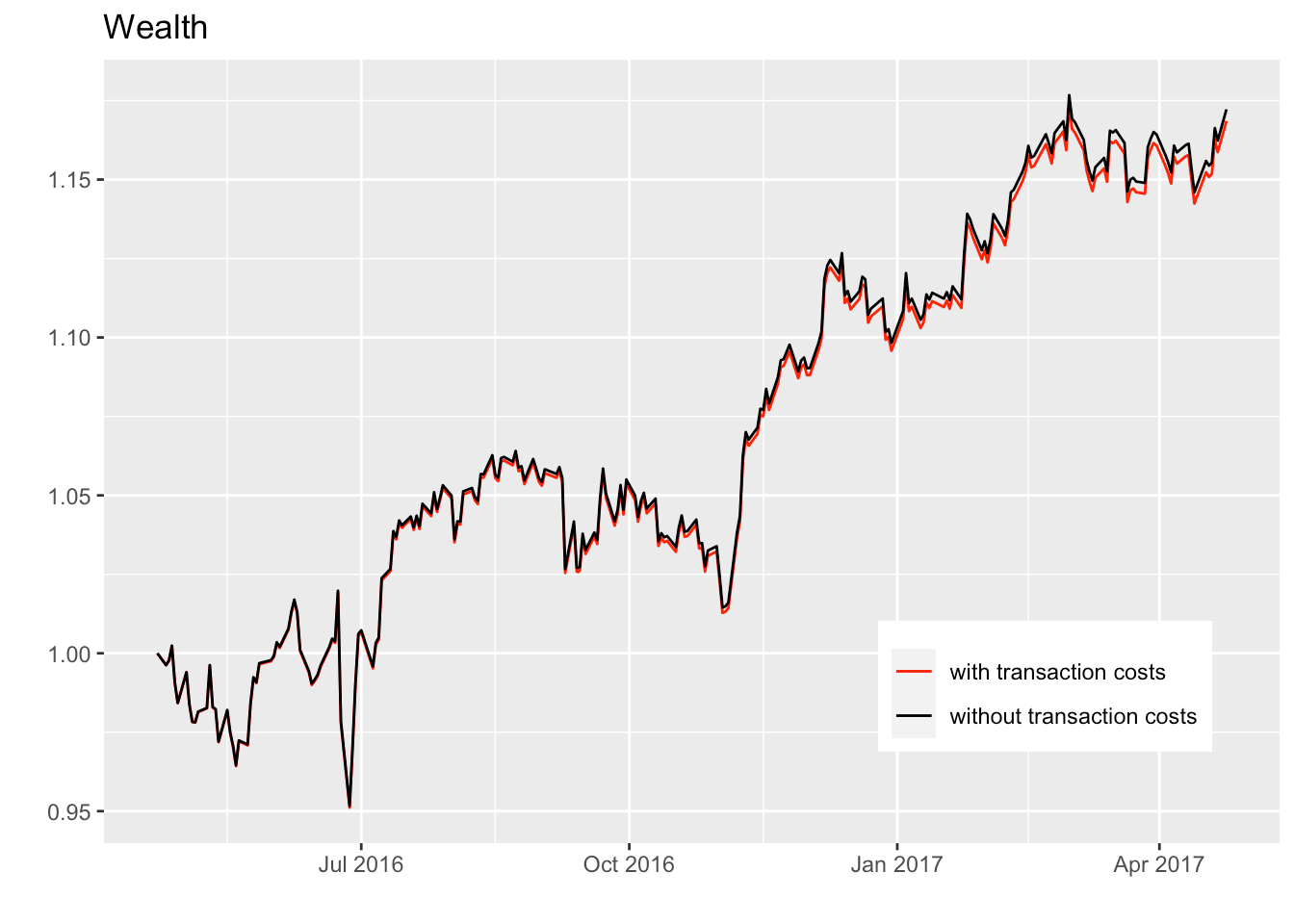## Incorporating benchmarks

When performing the backtest of the designed portfolio functions, one may want to incorporate some benchmarks. The package currently suppports two benchmarks: 1/N portfolio and index of the market. (Note that to incorporate the index benchmark each dataset needs to contain one xts object named index.) Once can easily choose the benchmarks by passing the corresponding value to argument benchmark:

bt <- portfolioBacktest(portfolios, dataset10, benchmark = c("1/N", "index"))
#> Backtesting 3 portfolios over 10 datasets (periodicity = daily data)...
#> Backtesting benchmarks...
names(bt)
#>  "Quintile"  "GMVP"      "Markowitz" "1/N"       "index"

## Parameter tuning in portfolio functions

Portfolio functions usually contain some parameters that can be tuned. One can manually generate different versions of such portfolio functions with a variety of parameters. Fortunately, the function genRandomFuns() helps with this task by automatically generating different versions of the portfolios with randomly chosen paramaters:

# define a portfolio with parameters "lookback", "quintile", and "average_type"
quintile_portfolio_fun <- function(dataset, ...) {
prices <- tail(dataset$adjusted, lookback) X <- diff(log(prices))[-1] mu <- switch(average_type, "mean" = colMeans(X), "median" = apply(X, MARGIN = 2, FUN = median)) idx <- sort(mu, decreasing = TRUE, index.return = TRUE)$ix
w <- rep(0, ncol(X))
w[idx[1:ceiling(quintile*ncol(X))]] <- 1/ceiling(quintile*ncol(X))
return(w)
}

# then automatically generate multiple versions with randomly chosen parameters
portfolio_list <- genRandomFuns(portfolio_fun = quintile_portfolio_fun,
params_grid = list(lookback = c(100, 120, 140, 160),
quintile = 1:5 / 10,
average_type = c("mean", "median")),
name = "Quintile",
N_funs = 40)
#> Generating 40 functions out of a total of 40 possible combinations.

names(portfolio_list[1:5])
#>  "Quintile (lookback=140, quintile=0.5, average_type=mean)"
#>  "Quintile (lookback=160, quintile=0.1, average_type=mean)"
#>  "Quintile (lookback=120, quintile=0.5, average_type=mean)"
#>  "Quintile (lookback=120, quintile=0.1, average_type=median)"
#>  "Quintile (lookback=120, quintile=0.4, average_type=median)"

portfolio_list[]
#> function(dataset, ...) {
#>   prices <- tail(dataset$adjusted, lookback) #> X <- diff(log(prices))[-1] #> mu <- switch(average_type, #> "mean" = colMeans(X), #> "median" = apply(X, MARGIN = 2, FUN = median)) #> idx <- sort(mu, decreasing = TRUE, index.return = TRUE)$ix
#>   w <- rep(0, ncol(X))
#>   w[idx[1:ceiling(quintile*ncol(X))]] <- 1/ceiling(quintile*ncol(X))
#>   return(w)
#> }
#> <environment: 0x7fe974c77290>
#> attr(,"params")
#> attr(,"params")$lookback #>  140 #> #> attr(,"params")$quintile
#>  0.5
#>
#> attr(,"params")$average_type #>  "mean" Now we can proceed with the backtesting: bt <- portfolioBacktest(portfolio_list, dataset10) #> Backtesting 40 portfolios over 10 datasets (periodicity = daily data)... Finally we can observe the performance for all combinations of parameters backtested: plotPerformanceVsParams(bt) #> Parameter grid: #> lookback = c(100, 120, 140, 160) #> quintile = c(0.1, 0.2, 0.3, 0.4, 0.5) #> average_type = c("mean", "median") #> #> Parameter types: 0 fixed, 2 variable numeric, and 1 variable non-numeric.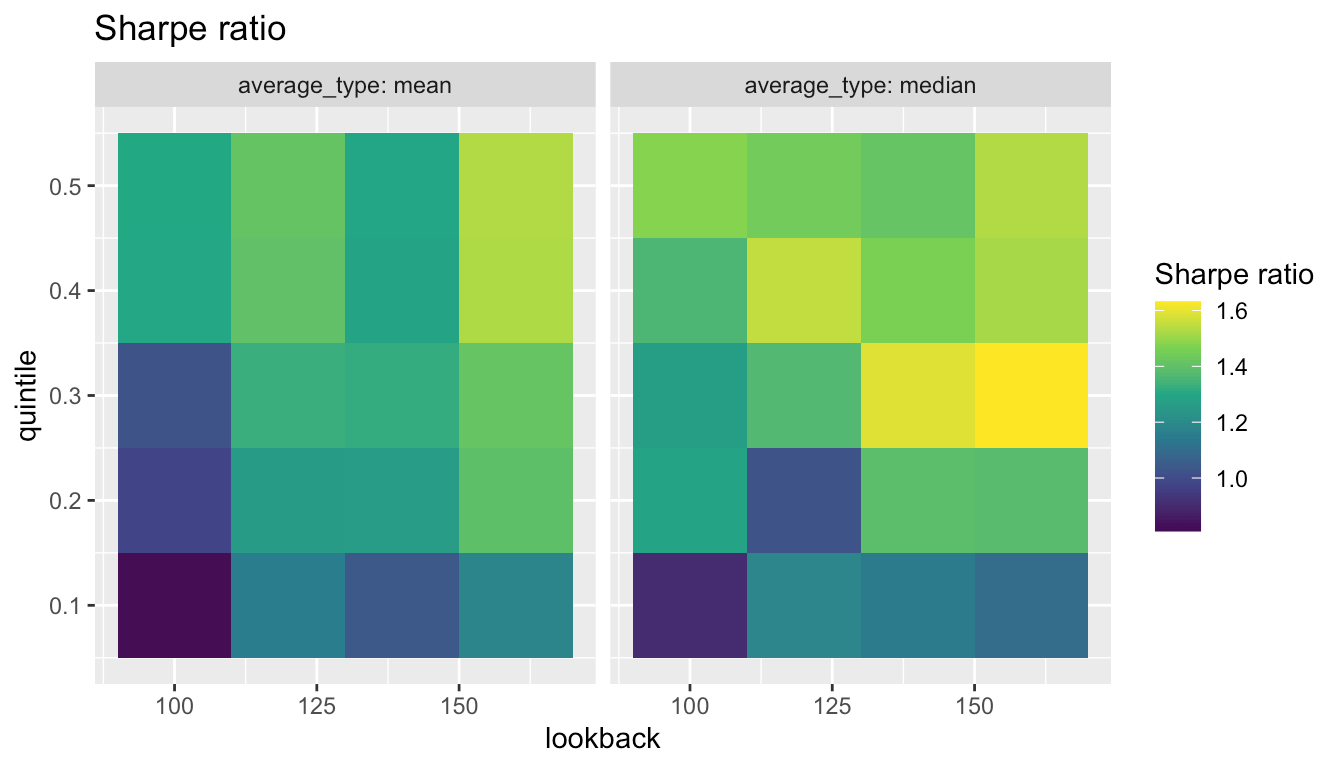In this case, we can conclude that the best combination is to use the median of the past 160 days and using the 0.3 top quintile. Extreme caution has to be taken when tuning hyper-parameter of strategies due to the danger of overfitting (Bailey et al. 2016). ## Progress bar In order to monitor the backtest progress, one can choose to turn on a progress bar by setting the argument show_progress_bar: bt <- portfolioBacktest(portfolios, dataset10, show_progress_bar = TRUE) ## Parallel backtesting The backtesting typically incurs in a very heavy computational load when the number of portfolios or datasets is large (also depending on the computational cost of each portfolio function). The package contains support for parallel computational mode. Users can choose to evaluate different portfolio functions in parallel or, in a more fine-grained way, to evaluate multiple datasets in parallel for each function: portfun <- Markowitz_portfolio_fun # parallel = 2 for functions system.time( bt_noparallel <- portfolioBacktest(list(portfun, portfun), dataset10) ) #> Backtesting 2 portfolios over 10 datasets (periodicity = daily data)... #> user system elapsed #> 63.594 0.730 65.451 system.time( bt_parallel_funs <- portfolioBacktest(list(portfun, portfun), dataset10, paral_portfolios = 2) ) #> Backtesting 2 portfolios over 10 datasets (periodicity = daily data)... #> user system elapsed #> 0.689 0.201 38.423 # parallel = 5 for datasets system.time( bt_noparallel <- portfolioBacktest(portfun, dataset10) ) #> Backtesting 1 portfolios over 10 datasets (periodicity = daily data)... #> user system elapsed #> 31.136 0.300 31.862 system.time( bt_parallel_datasets <- portfolioBacktest(portfun, dataset10, paral_datasets = 5) ) #> Backtesting 1 portfolios over 10 datasets (periodicity = daily data)... #> user system elapsed #> 1.538 0.377 21.089 It is obvious that the evaluation time for backtesting has been significantly reduced. Note that the parallel evaluation elapsed time will not be exactly equal to the original time divided by parallel cores because starting new R sessions also takes extra time. Besides, the two parallel modes can be simultaneous used. Note that an unexpected error might be thrown out when running a parallel backtest through RStudio in macOS. If that happens, one can check the default parallel setting via: parallel:::getClusterOption("setup_strategy") If "parallel" is returned, one can set the option setup_strategy to "sequential": parallel:::setDefaultClusterOptions(setup_strategy = "sequential") The problem may be fixed. However, the “sequential” strategy might be less efficient than the “parallel” strategy. ## Initialization for each backtest In some cases, one may want to do initialize some variable at the beginning of each backtest and be able to access those variables during the rolling-window process. At the moment, the package does not support this initialization. However, there is a hack that can be used for the time being (via the use of non-recommended global variables): allocation <- 0 # initialize global variable to 0 test_portfolio <- function(dataset, ...) { N <- ncol(dataset$adjusted)

w <- rep(allocation, N)
allocation <<- 1/N  # after first time it becomes 1/N

return(w)
}

bt <- portfolioBacktest(list("test" = test_portfolio),
dataset_list = dataset10[1:2],
lookback = 100, optimize_every = 200,
paral_datasets = 2)  # <--- this argument is necessary (has to be > 1)
#> Backtesting 1 portfolios over 2 datasets (periodicity = daily data)...

# sanity check
bt$test$dataset 1$w_optimized[, 1:2] #> MAS MGM #> 2015-09-15 0.00 0.00 #> 2016-06-30 0.02 0.02 #> 2017-04-18 0.02 0.02 bt$test$dataset 2$w_optimized[, 1:2]
#>             XRX ULTA
#> 2014-03-04 0.00 0.00
#> 2014-12-16 0.02 0.02
#> 2015-10-02 0.02 0.02

Note that for this hack to work, one needs paral_datasets > 1.

## Tracing where execution errors happen

Execution errors during backtesting may happen unexpectedly when executing the different portfolio functions. Nevertheless, such errors are properly catched and bypassed by the backtesting function portfolioBacktest() so that the execution of the overall backtesting is not stopped. For debugging purposes, to help the user trace where and when the execution errors happen, the result of the backtesting contains all the necessary information about the errors, including the call stack when a execution error happens. Such information is given as the attribute error_stack of the returned error_message.

For example, let’s define a portfolio function that will throw a error:

sub_function2 <- function(x) {
"a" + x  # an error will happen here
}

sub_function1 <- function(x) {
return(sub_function2(x))
}

wrong_portfolio_fun <- function(data, ...) {
N <- ncol(data$adjusted) uni_port <- rep(1/N, N) return(sub_function1(uni_port)) } Now, let’s pass the above portfolio function to portfolioBacktest() and see how to check the error trace: bt <- portfolioBacktest(wrong_portfolio_fun, dataset10) #> Backtesting 1 portfolios over 10 datasets (periodicity = daily data)... res <- backtestSelector(bt, portfolio_index = 1) # information of 1st error error1 <- res$error_message[]
str(error1)
#>  chr "non-numeric argument to binary operator"
#>  - attr(*, "error_stack")=List of 2
#>   ..$at : chr "\"a\" + x" #> ..$ stack: chr "sub_function1(uni_port)\nsub_function2(x)"

# the exact location of error happening
cat(attr(error1, "error_stack")$at) #> "a" + x # the call stack of error happening cat(attr(error1, "error_stack")$stack)
#> sub_function1(uni_port)
#> sub_function2(x)

## Backtesting over files: usage for grading students

In some situations, one may have to backtest portfolios from different sources stored in different files, e.g., students in a porftolio design course (in fact, this package was originally developed to assess students in the course “Portfolio Optimization with R” from the MSc in Financial Mathematics (MAFM)). In such cases, the different portfolios may have conflicting dependencies and loading all of them into the environment may not be a reasonable approach. The package adds support for backtesting portfolios given in individual files in a folder in a way that each is executed in a clean environment without affecting each other. It suffices to write each portfolio function into an R script (with unique filename) containing the portfolio function named exactly portfolio_fun() as well as any other auxiliary functions that it may require (needless to say that the required packages should be loaded in that script with library()). All theses files should be put into a file folder, whose path will be passed to the function portfolioBacktest() with the argument folder_path.

If an instructor wants to evaluate students of a course in their portfolio design, this can be easily done by asking each student to submit an R script with a unique filename like STUDENTNUMBER.R. For example, suppose we have three files in the folder portfolio_files named 0001.R, 0002.R, and 0003.R. Then:

bt_all_students <- portfolioBacktest(folder_path = "portfolio_files",
source_to_local = FALSE,
dataset_list = dataset10)
#> Backtesting 3 portfolios over 10 datasets (periodicity = daily data)...
names(bt_all_students)
#>  "0001" "0002" "0003"

Note that if the package CVXR is used in some of the files, it may not work depending on the version. A temporary workaround is to set the argument source_to_local = FALSE in portfolioBacktest() (the side effect is that the objects from the file will be loaded in the global environment).

## Leaderboard of portfolios with user-defined ranking

Now we can rank the different portfolios/students based on a weighted combination of the rank percentiles (termed scores) of the performance measures:

leaderboard <- backtestLeaderboard(bt_all_students,
weights = list("Sharpe ratio"  = 7,
"max drawdown"  = 1,
"annual return" = 1,
"ROT (bps)"     = 1))

library(gridExtra)
grid.table(leaderboard$leaderboard_scores)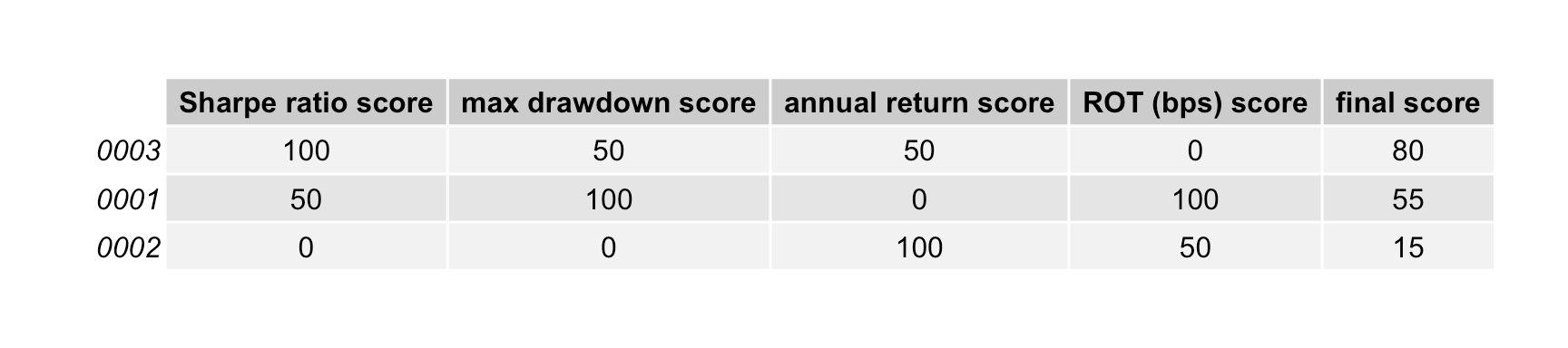## Example of a script file to be submitted by a student Consider the student with id number 666. Then the script file should be named 666.R and should contain the portfolio function called exactly portfolio_fun() as well as any other auxiliary functions that it may require (and any required package loading with library()): library(CVXR) auxiliary_function <- function(x) { # here whatever code } portfolio_fun <- function(data, ...) { X <- as.matrix(diff(log(data$adjusted))[-1])  # compute log returns
mu <- colMeans(X)  # compute mean vector
Sigma <- cov(X)  # compute the SCM
# design mean-variance portfolio
w <- Variable(nrow(Sigma))
prob <- Problem(Maximize(t(mu) %*% w - 0.5*quad_form(w, Sigma)),
constraints = list(w >= 0, sum(w) == 1))
result <- solve(prob)
return(as.vector(result\$getValue(w)))
}

# Appendix

## Performance criteria

The performance criteria currently considered by default in the package are:

• annual return: the (geometric) annualized return;
• annual volatility: the annualized standard deviation of returns;
• max drawdown: the maximum drawdown is defined as the maximum loss from a peak to a trough of a portfolio;
• Sharpe ratio: the annualized Sharpe ratio, the ratio between the (geometric) annualized return and the annualized standard deviation;
• Sterling ratio: the return over average drawdown, see here for complete definition. In the package, we use $\text{Sterling ratio} = \frac{\text{annualized return}}{\text{max drawdown}};$
• Omega ratio: the probability weighted ratio of gains over losses for some threshold return target, see here for complete definition. The ratio is calculated as: $\Omega(r) = \frac{\int_{r}^{\infty} (1-F(x))dx}{\int_{-\infty}^{r} F(x)dx};$ In the package, we use $$\Omega(0)$$, which is also known as Gain-Loss-Ratio.
• ROT bps: Return over Turnover (ROT) defined as the sum of cummulative return over the sum of turnover.

One can easily add new performance measures with the function add_performance().